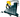# Fuel Consumption Calculator

Distance Driven

km

Fuel Consumed

L

Cost per Unit of Fuel

\$### Fuel Consumption

Fuel consumption is the quantity of fuel used per unit length, (liters per 100 kilometers (L/100 km)).

### Fuel Consumption Formula

Divide the liters it took to refill the tank by the distance traveled and multiply by 100.
example: 60 litres / 800 km x 100 = 7.5 litres per 100km (expressed 7.5L/100km).
To Calculate Kilometres per liter fuel economy?
Divide the kilometers traveled by the number of liters it took to refill the tank.
example: 800km / 60 litres = 13.3km per litre